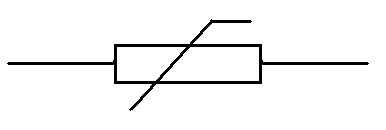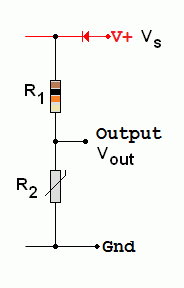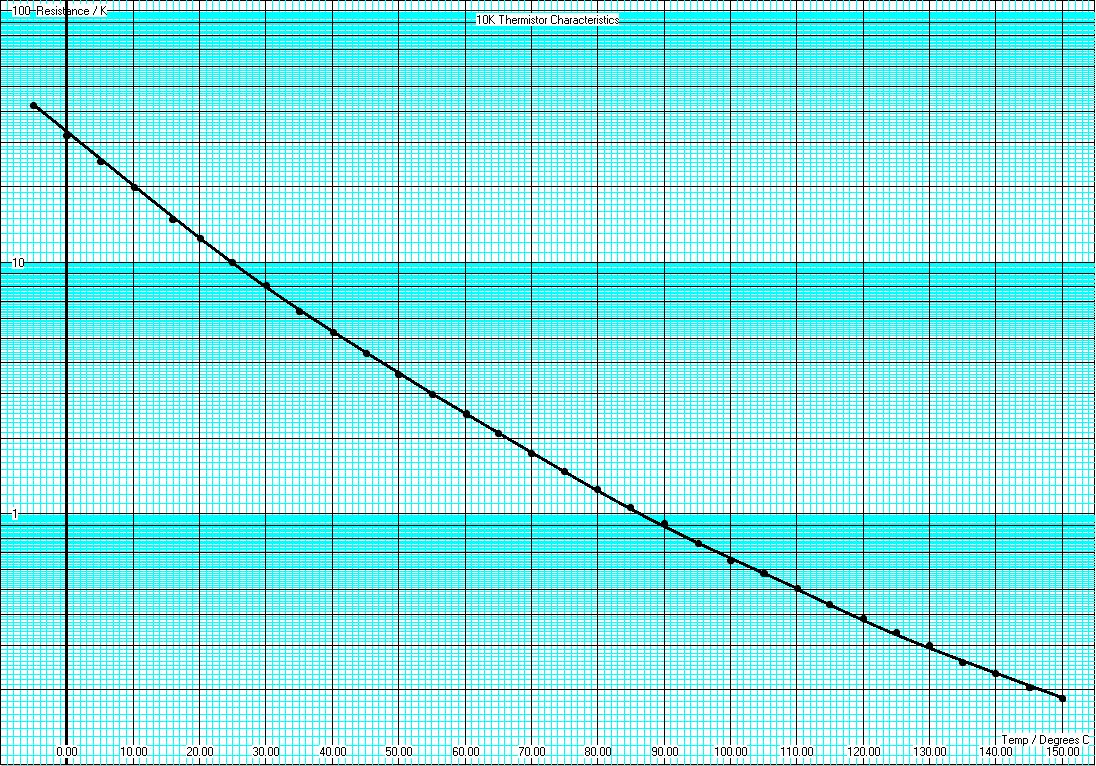RANDOM PAGE

SITE SEARCH

LOG
IN

HELP

# Thermistor

This is the AQA version closing after June 2019. Visit the the version for Eduqas instead.

## Temperature Dependent Resistor (TDR)• Negative temperature coefficient resistors (NTC Resistors)
• As the temperature rises, the thermistor resistance drops.
• At low temperatures, few electrons are free to move in the semiconductor material.
• As the temperature rises, increased thermal vibrations cause more electrons to break free and flow.
• Thermistors are used to measure temperature.
• They are fairly slow to react.
• Measurement circuits must be designed with care because the current flowing in the TDR must not significantly heat it up.
• Note that devices like incandescent bulbs behave in the opposite way. They increase their resistance as the temperature rises. This is a positive temperature coefficient.

## Thermistor Voltage Divider• Vout = Vs x R2 / (R1 + R2)

## Log Graphs

The thermistor resistance changes over such a large range that normal graphs are not useful. Log graphs solve the problem. Here is an example based on the data sheet for a thermistor with 10K at 25 C.• At 50 the resistance is 40kΩ
• At 250 the resistance is 200Ω
• When the resistance is 3kΩ the temperature is just under 150
• When the resistance is 50Ω the temperature is 300

## Calculating a Safe Series Resistor

If possible, three design aims need to be met.

1. The current through the thermistor must be small so it does not heat up and alter the temperature you are trying to measure. (Alternatively, the circuit could be turned on for a short time so the thermistor does not have time to heat up.)
2. When the thermistor gets hot, its resistance drops close to zero. Under these conditions, the current must not exceed the maximum safe value for the thermistor.
3. The series resistor value should be similar to the thermistor resistance at the temperature we are trying to detect.

For example in a refrigerator, the cooling pump might come on if the temperature rises above 5 Celsius. From the graph above, the thermistor resistance is about 40kΩ. A 40kΩ series resistor could be used. In a 12 volt circuit, at 50, the current flowing would be ...

I = V / R

I = 12 / 80x103

I = 0.15 mA

This tiny current would not have much of a heating effect.

The series resistor is similar in value to the thermistor at 50C.

At much higher temperatures the current would still be less that 0.3mA.